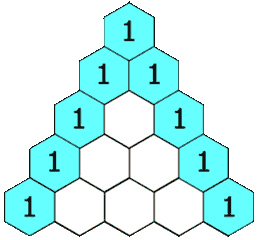Medium

# Get specific row K in a Pascal Triangle

Given a row index K, write a program to print the Kth of Pascal triangle.

Note: row index starts from 0.

Pascal Triangle:Note: In Pascal's triangle, each number is the sum of the two numbers directly above it. (Image reference: Wiki)

Example:

```K = 2
Output: 1, 1

K= 5
Output: 1, 4, 6, 4, 1
```

Simple Approach:

As we discussed here - Pascal triangle, starting calculating the rows from 1 to K and then print the Kth row.

Output:

```[1, 7, 21, 35, 35, 21, 7, 1]
```

Better Solution:

We do not need to calculate all the k rows to know the kth row. We can find the pattern followed in all the rows and then use that pattern to calculate only the kth row and print it.

Pattern: Let's take K = 7

```Output: 1, 7, 21, 35, 35, 21, 7, 1

Index 0 = 1
Index 1 = 7/1 = 7
Index 2 = 7x6/1x2 = 21
Index 3 = 7x6x5/1x2x3 = 35
Index 4 = 7x6x5x4/1x2x3x4 = 35
Index 5 = 7x6x5x4x3/1x2x3x4x5 = 21
Index 6 = 7x6x5x4x3x2/1x2x3x4x5x6 = 21
Index 7 = 7x6x5x4x3x2x1/1x2x3x4x5x6x7 = 1
```

Have start=1, end = 7. So take a variable current = 1. Iterate from i = 1 to 7 and for each iteration do current = current * end and then current = current / start, then current will be ith element of the row. Do end-- and start++.

Output:

```[1, 7, 21, 35, 35, 21, 7, 1]
```

Reference: Leetcode